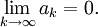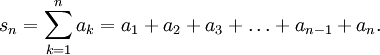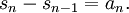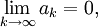# A Useful Theorem

Previous: Introduction to the Divergence Test

Next: The Divergence Test

The following theorem will yield the divergence test.

Theorem 1

If the infinite seriesis convergent, then### Proof of Theorem 1

The proof of this theorem can be found in most introductory calculus textbooks that cover the divergence test and is supplied here for convenience. Let the partial sumbeThenandBy assumption, an is convergent, so the sequence {sn} is convergent (using the definition of a convergent infinite series). Let the number S be given bySince n-1 also tends to infinity as n tends to infinity, we also haveFinally,Thus, ifis convergent, thenas required.

Previous: Introduction to the Divergence Test

Next: The Divergence Test